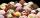Party

At the party everyone clink with everyone. Together, they clink 406 times. How many people were at the party?

Result

On party was:  29 people

Solution:Leave us a comment of example and its solution (i.e. if it is still somewhat unclear...):Be the first to comment!To solve this example are needed these knowledge from mathematics:

Looking for help with calculating roots of a quadratic equation? Would you like to compute count of combinations?

Next similar examples:

1. ChordsHow many 4-tones chords (chord = at the same time sounding different tones) is possible to play within 7 tones?
2. CombinationsFrom how many elements we can create 990 combinations 2nd class without repeating?
3. CombinationsHow many elements can form six times more combinations fourth class than combination of the second class?
4. ConfectioneryThe village markets have 5 kinds of sweets, one weighs 31 grams. How many different ways a customer can buy 1.519 kg sweets.
5. BlocksThere are 9 interactive basic building blocks of an organization. How many two-blocks combinations are there?
6. ExaminationThe class is 21 students. How many ways can choose two to examination?
7. TeamsHow many ways can divide 16 players into two teams of 8 member?
8. TrinityHow many different triads can be selected from the group 43 students?
9. Fish tankA fish tank at a pet store has 8 zebra fish. In how many different ways can George choose 2 zebra fish to buy?
10. The confectioneryThe confectionery sold 5 kinds of ice cream. In how many ways can I buy 3 kinds if order of ice creams does not matter?
11. Calculation of CNCalculate: ?
12. DiscriminantDetermine the discriminant of the equation: ?
13. Theorem proveWe want to prove the sentence: If the natural number n is divisible by six, then n is divisible by three. From what assumption we started?
14. EquationEquation ? has one root x1 = 8. Determine the coefficient b and the second root x2.Find the roots of the quadratic equation: 3x2-4x + (-4) = 0.Determine the quadratic equation absolute coefficient q, that the equation has a real double root and the root x calculate: ?If 5x + x² > 100, then x is not